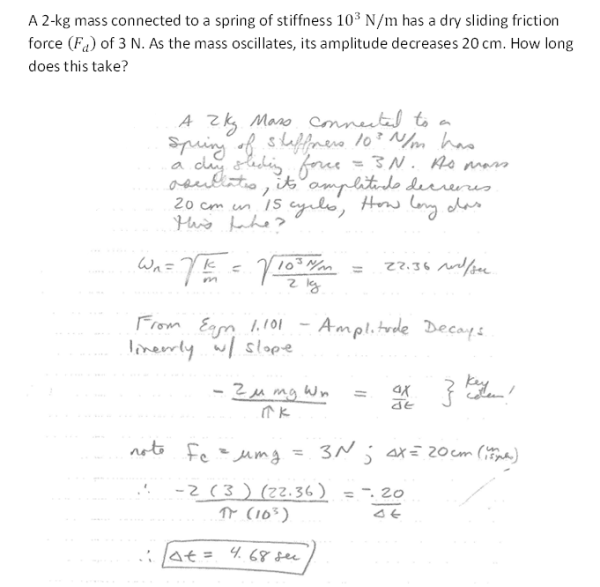A 2-kg mass connected to a spring of stiffness 10^3 N/m has a dry sliding friction force (Fd) of 3 N. As the mass oscillates, its amplitude decreases 20 cm. How long does this take?A 2-kg mass connected to a spring of stiffness 10^3 N/m has a dry sliding friction force (Fd) of 3 N. As the mass oscillates, its amplitude decreases 20 cm. How long does this take?

Vibrations Page 1 vibrations vibrations vibrations vibrations vibrations vibrations vibrations Vibrations Page 2